# CAT 2017 – Slot 1 – Quantitative Ability – For how many integers n, will the inequality (n – 5) (n – 10) – 3(n – 2) ≤ 0 be satisfied

Q. 1: For how many integers n, will the inequality (n – 5) (n – 10) – 3(n – 2) ≤ 0 be satisfied?

On solving the equation, we get n^2 – 18n + 56 ≤ 0

Factorize and we get, (n-4)(n-14) ≤ 0

4 ≤ n ≤ 14

No of values of n =11

##### Q. 2: If f1(x) = x^2 + 11x + n and f2(x) = x, then the largest positive integer n for which the equation f1(x) = f2(x) has two distinct real roots, is

f1(x) = f2(x)

x^2 + 11x +n = x

x^2 + 10x + n =0

To have distinct and real roots, D>0

D = b^2-4ac = 100 – 4n > 0

On solving the inequality, we get, n<25

So, max positive integer value of n = 24.

Q. 3: If a, b, c, and d are integers such that a + b + c + d = 30, then the minimum possible value of (a – b)^2 + (a – c)^2 + (a – d)^2 is

a + b + c + d = 30

a, b, c, d are integers. (a – b)^2 + (a – c)^2 + (a – d)^2 would have its minimum value when each bracket has the least possible value. Let (a, b, c, d) = (8, 8, 7, 7) The given expression would be 2. It cannot have a smaller value.

Q. 4: Let AB, CD, EF, GH, and JK be five diameters of a circle with center at O. In how many ways can three points be chosen out of A, B, C, D, E, F, G, H, J, K, and O so as to form a triangle?

There are 11 points from which a triangle can be formed. But there are 5 lines which have 3 points linearly.

Number of triangles formed = 11C3 – 5 (because of the lines)

165 – 5 = 160 triangles

Q. 5: The shortest distance of the point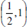from the curve y = |x -1| + |x + 1| is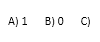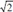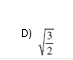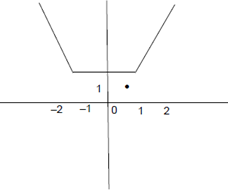Explanation:-

The graph of y = |x – 1| + |x + 1| is shown above.

The shortest distance of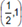from the graph is 1.
##### Checkout Other Questions of CAT 2017 Slot 1 Paper:

Verbal Ability :              |   Q.01- Q.06  |  Q.07- Q.12  |  Q.13- Q.18  |  Q.19- Q.21  |  Q.22- Q.24  |  Q.25- Q.29  |  Q.30 – Q.34  |

Logical Reasoning :    |   Q.29 – Q.32  |

Quantitative Aptitude: |

### Free Material Area